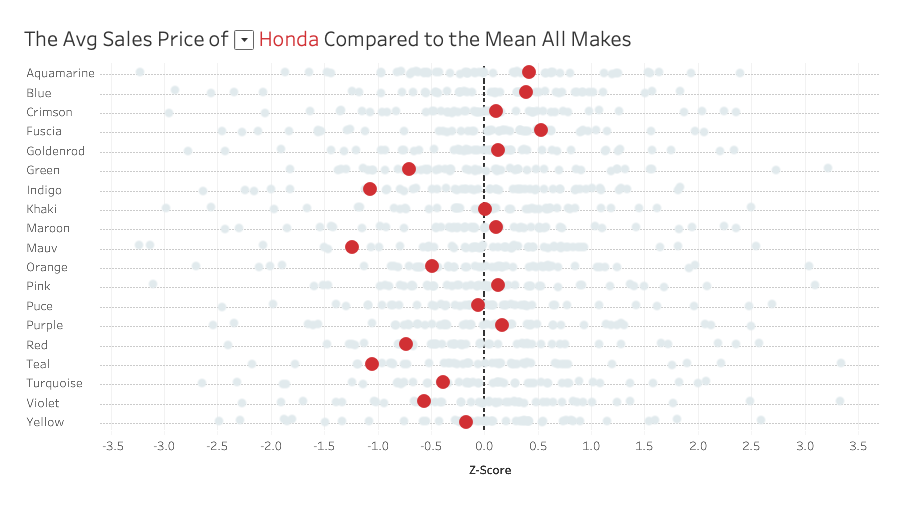## VizWiz

Launch, grow, and unlock your career in data

# How to Calculate a Z-ScoreA Z-score is a numerical measurement that describes a value's relationship to the mean of a group of values. Z-score is measured in terms of standard deviations from the mean.

• If a Z-score is 0, it indicates that the data point's score is identical to the mean score.
• A Z-score of 1.0 would indicate a value that is one standard deviation from the mean.
• Z-scores may be positive or negative, with a positive value indicating the score is above the mean and a negative score indicating it is below the mean.

The calculation you need is Tableau is:

( SUM([Profit]) - WINDOW_AVG(SUM([Profit])) )
/
WINDOW_STDEV(SUM([Profit]))

Replace the SUM([Profit]) with whichever measure you'd like to use at the aggregation that makes sense in your data.

Get the data used in the video here - https://data.world/vizwiz/car-sales-mock-data